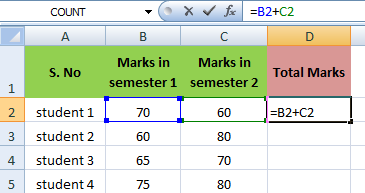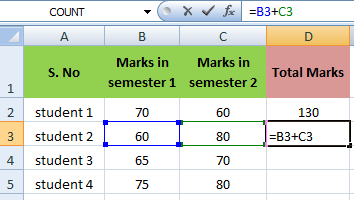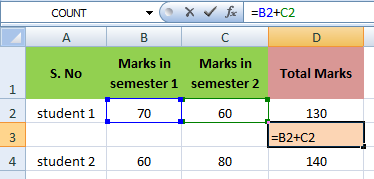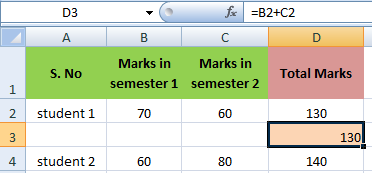### Copy Exact Formula in MS Excel

How to Copy Exact Formula in MS Excel
When you copy a formula in MS Excel, Cell References are automatically adjusted for each new cell the formula is copied to.
For example, cell D2 below contains a formula which adds the value of cell B2 and Cell C2.When you copy this formula to cell D3, the formula will automatically take the reference values.Now, If you want to copy the exact formula without changing the cell references, take following steps.
Let's learn with Example
>> Click in the formula bar and select the formula.
>> Press CTRL+c, and press Enter.
>> Select cell where you want to copy the formula and click in the formula bar.
>> Press CTRL+v, and press Enter.
Result will be:
Both cell D2 and D3 contain the exact same formula now.